Courses

# In some code, letters a, b, c, d and e represent numbers 2, 4, 5, 6 and 10. We just do not know which letter represents which number. Consider the following relationships:I. a + c = e,II. b – d = d andIII. e + a = bWhich of the following options are true?a)b = 4, d = 2b)a = 4, e = 6c)b = 6, e = 2d)a = 4, c = 6Correct answer is option 'B'. Can you explain this answer?

### Related TestBakliwal Institute Sep 16, 2021
We have a + c = e so possible summation 6+4=10 or 4+2 = 6.
Also b = 2d so possible values  4 = 2 * 2 or 10 = 5 * 2.
So considering both we have b = 10 , d = 5, a= 4 ,c = 2, e = 6.
Hence the correct option is B .Join EduRev Infinity today with great offers Get unlimited access to notes, videos & tests. Join discussion groups and get your doubts solved by teachers and fellow aspirants.

### Top Courses for CAT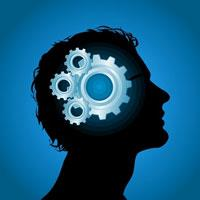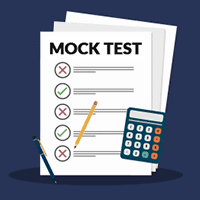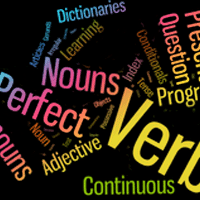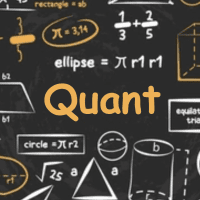### Related Content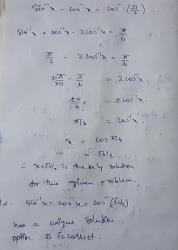Answer is option BDoc | 1 PageAnswer is option 'B'Doc | 1 Page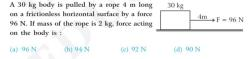Plz explainDoc | 1 Page